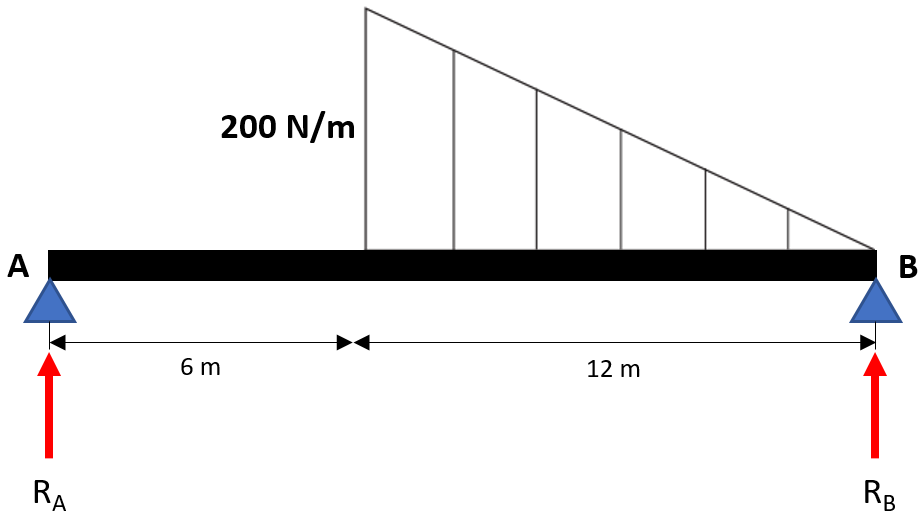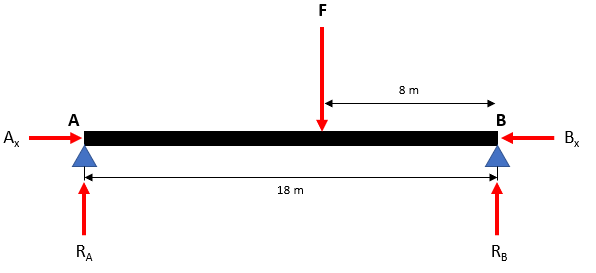## Reaction Point

Beam AB, which is simply supported at Points A & B, is subjected to a distributed load as shown. If the beam’s weight is negligible, what is the reaction force at Point A?Hint
Draw the free body diagram:Hint 2
Take the moment about Point B to reduce the amount of unknown forces.
$$\sum M_B=0=Force \times Distance$$$First, draw the free body diagram:The triangular force distribution can be replaced with a concentrated force $$F$$ , which is located through the triangle’s centroid. The force’s magnitude is equal to the triangle’s area: $$F=\frac{1}{2}bh=\frac{1}{2}(12m)(200N/m)=1,200\:N$$$
where $$b$$ is the triangle’s base, and $$h$$ is the triangle’s height.
We can take the moment about Point B to reduce our unknown variables down to 1 ( $$A_x$$ , $$B_x$$ and $$R_B$$ will zero out since their radius vector goes through Point B). Remember, $$Moment=Force \times Distance$$ :
$$\sum M_B=0=(18m)R_A-(8m)F$$$$$(18m)R_A=(8m)(1,200N)$$$
$$R_A=\frac{(8m)(1,200N)}{18m}=\frac{9,600N}{18}=533.3\:N$$\$
533.3 N